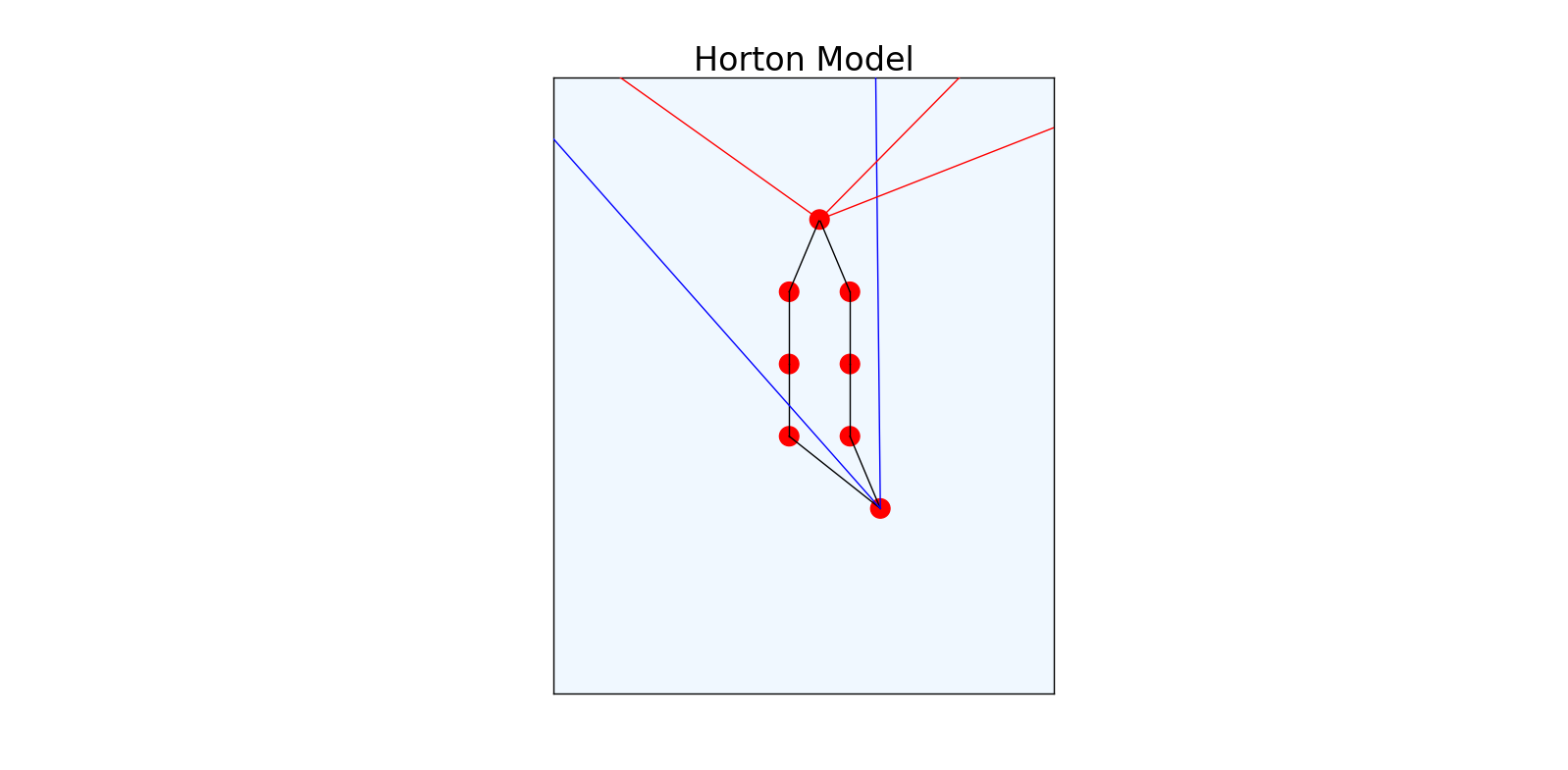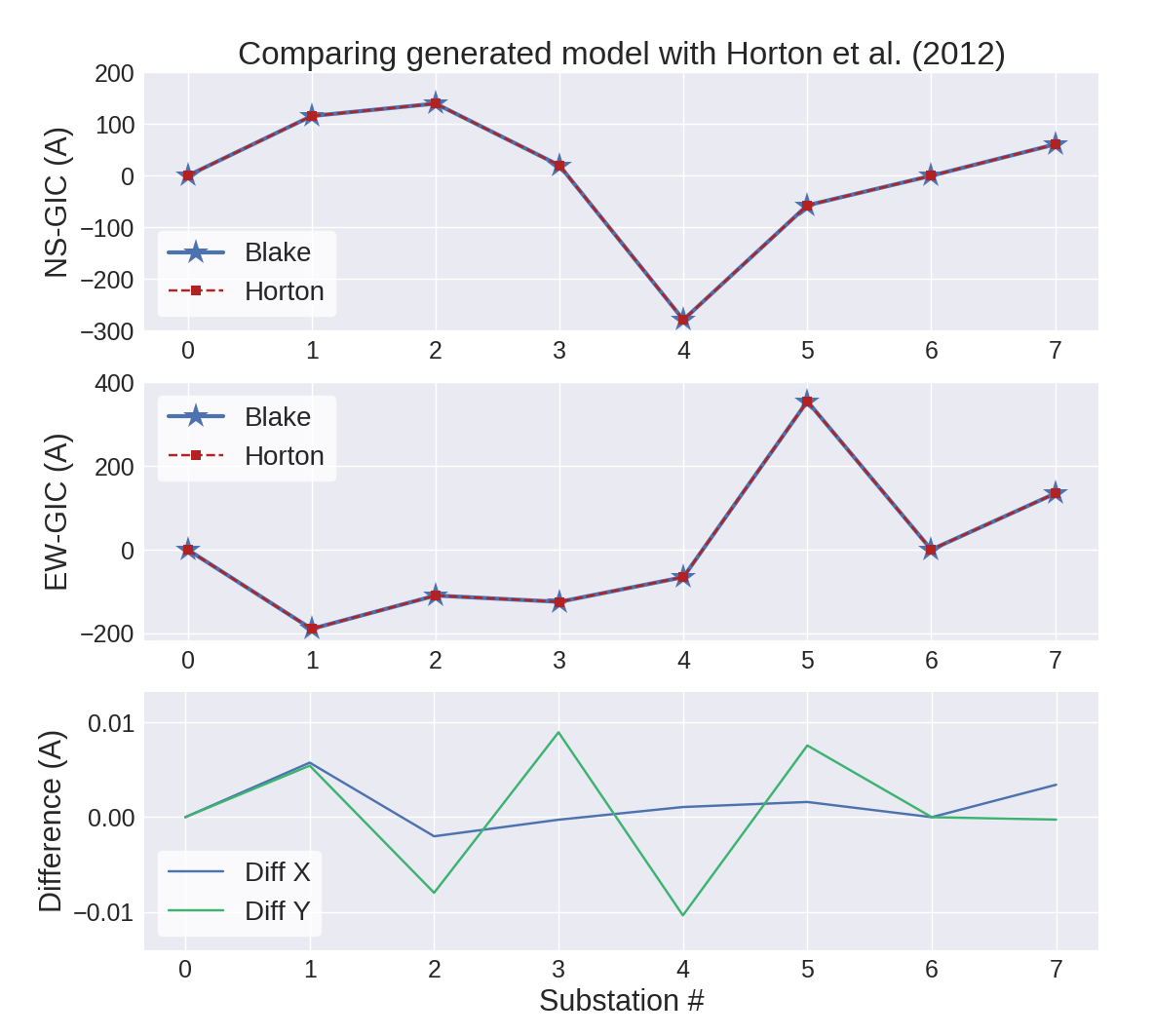# power-network-model Release 0.8

Python module to generate power network model for GIC calculations.

Keywords
geomagnetic, space, weather, GICs
MIT
Install
``` pip install power-network-model==0.8 ```

# Power_Network_ModelPython program to generate power network model for calculating geomagnetically induced currents.

The program handles different types of transformers (Auto and two-winding), and multiple transformers per substation. It connects correctly between voltage systems. In addition, parallel connections between substations are handled.

Uses the approach outlined here.

This code appears in a manuscript currently submitted to Space Weather. Links to completed paper to follow.

## Installation

`pip install power_network_model`

## Quick Code Example

If you have two .csv files with network information- one for connections, one for transformers:

```import pkg_resources
import power_network_model as PNM
import power_network_model.plotter as PNMplot

# read in transformer file, generate substation data:
trafo_filename = pkg_resources.resource_filename("power_network_model", 'Data/Horton_Input_Trafos.csv')
ss_trafos, ss_connections, ss_meta = PNM.make_substations(trafo_filename)

# Generate connections between substations:
connections_filename = pkg_resources.resource_filename("power_network_model", 'Data/Horton_Input_Connections.csv')

# Write out the output files
filename = "Horton_Output"
nodes_output, connections_output = PNM.write_out(filename, ss_trafos, ss_connections, connections_twixt_stations)

################################################################################
# If you want to plot the output:
PNMplot.network_plotter(nodes_output, connections_output, ["red", "blue"], "Horton Model")```

The above gives you the following model:The connections at one substation in this model are shown here:With red, blue and black lines for 500 kV, 345 kV and internal connections respectively. This substation connects two voltage regimes, and has two YY-transformers (3 nodes apiece).

## Inputs Required

The two .csv file inputs need to be in the following format:

Transformer information in a csv file with columns:

• SS_NAME = Subsation name (important to keep spelling uniform)
• SS_SYM = Substation symbol
• TF_SYM = Transformer symbol (or code: e.g, T1432)
• VOLTAGE = Highest operating voltage of the substation
• LAT = Latitude
• LON = Longitude
• TYPE = Type of transformer: 'A' = autotransformer, "YY" = Wye-Wye, "G" = grounded, "T" = Tee connection station.
• RES1 = High voltage resistance winding
• RES2 = Low voltage resistance winding
• GROUND = Ground resistance at substation
• SWITCH = Transformer ground switch: 0 = None, 1 = Open, 2 = Closed

Connections information in a csv file with columns:

• FROM = Substation name from
• TO = Substation name to
• VOLTAGE = Voltage of connection
• CIRCUIT = To denote multiple lines per connection
• RES = Resistance of line.

## Output Files

Two .txt files are output, one for node information, and one for connections.

Nodes file has columns (left-to-right):

• Node number
• Substation latitude
• Substation longitude
• Transformer winding resistance
• Grounding resistance
• Voltage of node
• Substation ID
• Transformer ID
• Real (0) or imaginary (nan) node

Connections file has columns (left-to-right):

• Number of node from
• Number of node to
• Resistance of connection
• Latitude of node from
• Longitude of node from
• Latitude of node to
• Longitude of node to
• Dummy coluimn (with nans)
• Voltage of Line

## Verification

Verified with the Horton 2012 model (included in Data/). After calculating GICs for a uniform electric field, compared to Horton:Finally, when applied to a larger network such as Ireland:## Author

Written by Sean Blake in Trinity College Dublin, 2017

Email: blakese@tcd.ie